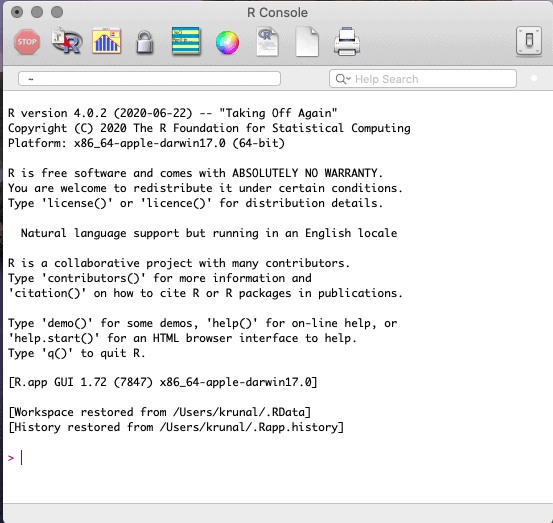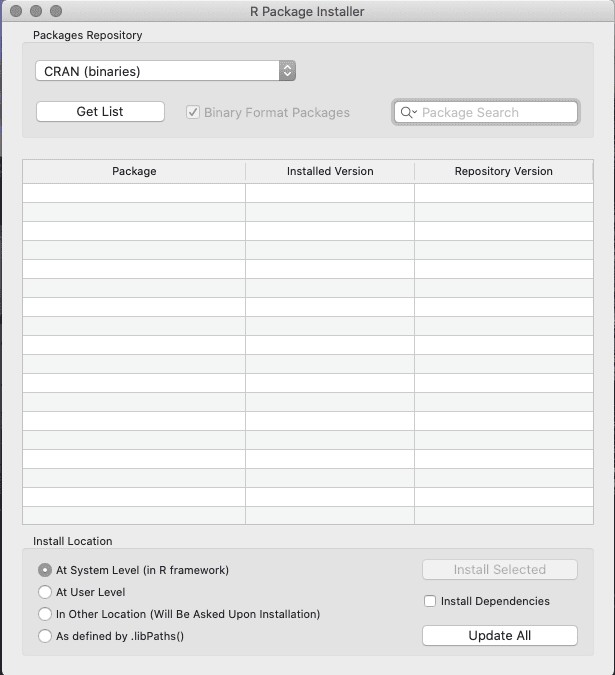# map() Function in R: How to Install Purrr Package

If you are working with functions and vectors, then you need to use the purrr package. The purrr package improves the R’s functional programming (FP) toolkit.

The map() function in R is categorized under functional programming that allows you to replace many for loops with code that is more concise and clearer to read.

## How to Install packages in R

But before using the map() function, we need to install the purrr package.

To install using GUI, open the R language application, and you will see the window like this.Click “Packages & Data” in the top menu, then click “Package Installer”.

Then you can choose the mirror that is closest to your geographical location.

And then you will see something like the below screen.Here, you can search for a purrr package or any package and tick on the Install Dependencies check box and install the package.

If there are still packages missing by any mistakes, you can install it one by one from here.

In R, if you want to install any third-party packages, you can download it from here, and then you can use it in your program.

Without purrr package, you will get the following error if you try to use the map() function.

``````Error in map(., function(x) x + 1) : could not find function "map"
Calls: %>% ... eval -> _fseq -> freduce -> withVisible -> <Anonymous>could not find function "%>%"``````

Means you got the `could not find function "map"` error.

To resolve could not find function “map” error, install and use the purrr package, and we have already seen how to install packages in R.

## R map

The map() function in R is used to apply a function to each Vector element. The map() methods(map and other variants) transform their input by applying a function to each item of a vector or list and returning the object which has the same length as the input.

### Syntax

``map(rv, func, ...)``

### Parameters

The rv is an atomic vector or list.

The func is a function, formula, or atomic vector.

1. If it is a function, it is used as it is.
2. If it is a formula, e.g., ~ .x + 1, it is converted to a function. There are three ways to refer to the arguments:

1. If it is a single argument function, use.
2. If there are two arguments function, use .x and .y.
3. It there are more arguments, use ..1, ..2, ..3 etc.

### Return Value

The map() function returns a vector the same length as input.

### Example of R map() on Vector

Pass a function in the argument, and map() function applies this function to all the vector elements.

``````# Pro.R

library(purrr)

rv <- 1:3
rv %>%
map(function(x) x + 1)``````

#### Output

``````[]
 2

[]
 3

[]
 4``````

First, we are importing the purrr package using the library() statement.

In the next step, we define a vector, and then we are incrementing each vector value by 1 using the map() function. You can see that the map() function applies +1 to every vector element, and it does not modify the length of the vector.

## Variants of map() functions in R

There are many variants of the map() functions available in R.

1. map_if(.rv, .p, .f, …)
2. map_at(.rv, .at, .f, …)
3. map_lgl(.rv, .f, …)
4. map_chr(.rv, .f, …)
5. map_int(.rv, .f, …)
6. map_dbl(.rv, .f, …)
7. map_dfr(.rv, .f, …, .id = NULL)
8. map_dfc(.rv, .f, …)

The rv is an input vector or list.

All the map variant functions return a vector the same length as an input.

The map_if() function is used to apply a function to only those items of the .rv that meet a specified condition.

The map_lgl() function returns a logical vector,

The map_int() function returns an integer vector.

The map_dbl() function returns a double vector.

The map_chr() function returns a character vector.

The map_dfr() and map_dfc() functions return a data frame created by row-binding and column-binding respectively. These functions are also required to install dplyr.

The output of .f will be automatically typed upwards, for example. logical -> integer -> double -> character.

## Applying map() function to list

To apply the same operations on all the list elements, use the map() function. It returns the transformed list of the same size as an input list.

``````library(purrr)

list_data <- list(19, 21, 46)
list_data %>%
map(function(x) x ^ 2)``````

#### Output

``````[]
 361

[]
 441

[]
 2116``````

We defined a list and used the map() function to transform every element into the input list elements’ squared.

## Compute normal distributions from an atomic vector

You can compute the normal distributions from the vector using the map() function. See the below code.

``````library(purrr)

1:10 %>%
map(function(x) rnorm(10, x))``````

#### Output

``````[]
 0.7827287 0.8524386 1.0590045 -1.2431661 0.4175271 1.4974232
 0.2870406 0.5992063 1.4848386 1.6218882

[]
 1.00669429 1.53255379 2.04919607 1.73777599 1.40305088 1.78990628
 0.72417310 0.68788464 0.73435835 -0.08438977

[]
 4.517348 4.074736 3.678984 1.685608 3.078273 4.279211 2.774305 2.994225
 4.006520 4.695956

[]
 2.365310 4.790439 4.514782 3.892882 2.655238 4.880776 3.393000 2.039758
 4.849628 2.765497

[]
 5.227805 7.523240 5.431224 3.455294 5.457139 3.887880 3.846306 5.197063
 5.574604 4.019978

[]
 7.095229 6.684091 4.094152 7.302912 8.209158 6.993704 5.047641 7.105118
 7.331152 5.688645

[]
 5.905737 5.314832 6.886748 8.110370 6.005540 5.490614 8.844083 7.163475
 5.294017 8.072895

[]
 8.455733 8.217845 8.385706 8.577377 7.520048 7.347701 6.831893 6.173750
 8.633676 8.496391

[]
 6.979906 8.384546 9.342730 9.802514 9.958217 8.582408 9.607740 8.294869
 9.111597 9.483675

[]
 9.229792 9.164576 9.420438 11.296276 9.494806 10.186833 10.290970
 9.919838 8.789936 10.279902``````

## Conclusion

R map() is an FP function used to apply a specific function to all the vector or list elements. It does not change the size of an input variable and returns the transformed data.

Categories R